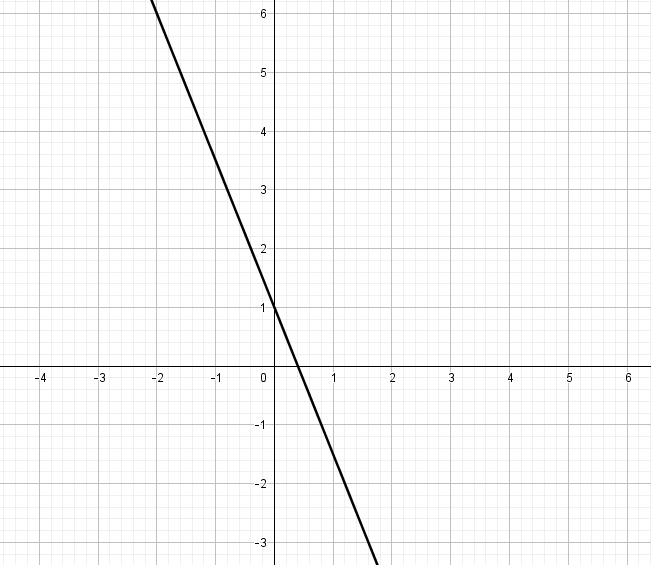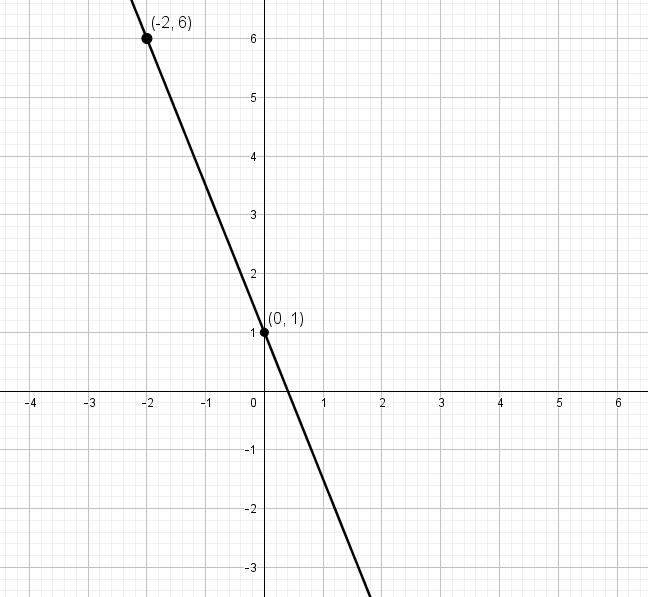# Using the slope formula to find the slope of a line

The “steepness” of a line in the xy-coordinate plane is called the slope. Slope can be positive (the line rises from left to right), negative (the line falls from left to right), zero (flat line), or even undefined (vertical line). In this lesson, we will look at how to calculate the slope using the slope formula and two points along a line.

## The slope formula

The slope of a line is often thought of as “rise over run”. This comes from the formula, which represents the change in y divided by the change in x. In other words, given two points, you will find the difference between the y-values and divide by the difference between the x-values.## Examples

To understand this formula, let’s look at a couple of examples. First, we will look at the case where you are simply given two points on a line and asked to find the slope. Then, we will see how to find the slope if you are just given the graph instead.

### Example (given two points)

Find the slope of a line that passes through the points $$(–1, 5)$$ and $$(1, 9)$$.

### Solution

Apply the slope formula by letting $$(x_1, y_1) = (–1, 5)$$ and $$(x_2, y_2) = (1, 9)$$.

\begin{align}\text{slope} &= \dfrac{y_2 – y_1}{x_2 – x_1} \\ &= \dfrac{9 – 5}{1 – (-1)} \\ &= \dfrac{4}{2} \\ &= \bbox[border: 1px solid black; padding: 2px]{2}\end{align}

Therefore, the slope is 2. Often, the slope is labelled m, so you could also write your answer as “$$m = 2$$”.

### Example (using the graph)

Find the slope of the line graphed below.### Solution

First, find two points on the line. You can pick any two points at all, so try to pick points that will be easy to work with or are easy to read from the graph.Now choose one point to be $$(x_1, y_1)$$ and the other to be $$(x_2, y_2)$$. It doesn’t matter which are which, as long as you are consistent with your choice in the formula. Here, we will let $$(x_1, y_1) = (–2, 6)$$ and $$(x_2, y_2) = (0, 1)$$.

\begin{align}\text{slope} = m &= \dfrac{y_2 – y_1}{x_2 – x_1}\\ &= \dfrac{1 – 6}{0 – (-2)}\\ &= \dfrac{-5}{2}\\ &= \bbox[border: 1px solid black; padding: 2px]{-\dfrac{5}{2}}\end{align}

## Other possibilities

It is possible for the slope of a line to be either undefined or to be zero. These are special cases, and explored more in the following lessons: